ZhouYongyi's Personal Blog# UIUC《文本检索与搜索引擎》课程学习笔记（三）—— 模型评估

## 引导问题（Guiding Questions）

Develop your answers to the following guiding questions while completing the readings and working on assignments throughout the week.

• Why is evaluation so critical for research and application development in text retrieval?
• How does the Cranfield evaluation methodology work?
• How do we evaluate a set of retrieved documents?
• How do you compute precision, recall, and F1?
• How do we evaluate a ranked list of search results?
• How do you compute average precision? How do you compute mean average precision (MAP) and geometric mean average precision (gMAP)?
• What is mean reciprocal rank?
• Why is MAP more appropriate than precision at k documents when comparing two retrieval methods?
• Why is precision at k documents more meaningful than average precision from a user’s perspective?
• How can we evaluate a ranked list of search results using multi-level relevance judgments?
• How do you compute normalized discounted cumulative gain (nDCG)?
• Why is normalization necessary in nDCG? Does MAP need a similar normalization? Why is it important to perform statistical significance tests when we compare the retrieval accuracies of two search engine systems?

• Mark Sanderson. Test collection based evaluation of information retrieval systems. Foundations and Trends in Information Retrieval 4, 4 (2010), 247-375.
• C. Zhai and S. Massung. Text Data Management and Analysis: A Practical Introduction to Information Retrieval and Text Mining, ACM Book Series, Morgan & Claypool Publishers, 2016. Chapter 9

## 关键概念（Key Phrases and Concepts）

Keep your eyes open for the following key terms or phrases as you complete the readings and interact with the lectures. These topics will help you better understand the content in this module.

• Cranfield evaluation methodology
• Precision and recall
• Average precision, mean average precision (MAP), and geometric mean average precision (gMAP)
• Reciprocal rank and mean reciprocal rank
• F-measure
• Normalized discounted cumulative Gain (nDCG)
• Statistical significance test

## 一、引言（Evaluation of TR System）

### 1.用什么指标来评估（What to Measure）

• 精度（Effectiveness/Accuracy）: 搜索结果的精度有多高？
-着眼于一个系统把相关文档排在无关文档前面的能力
• 效率（Efficiency）：用户多久才能得到结果？为了完成询问，需要多少的计算资源？
-着眼于时间、空间复杂度
• 适用性（Usability）：这个系统对于实际需求而言有帮助吗？
-进行用户调查

### 2.克兰菲尔德评估方法论（The Cranfield Evaluation Methodology）

• 1960年代诞生的一种用来选取实验检测中的要素的方法论
• 思想：构建一个可重用（reusable）的测试集&定义方法
– 一个文档样本集（模拟实际文档集）
– 一个关于主题/询问的样本集（模拟用户询问）
– 相关度判断（对于用户而言的理想结果）- 理想状态下的排名名单
• 一个能够重用的测试集，测试结果用来与其他系统对比

## 二、基本评估方法（Basic Measure）

### 1.准确率和召回率（Precision & Recall）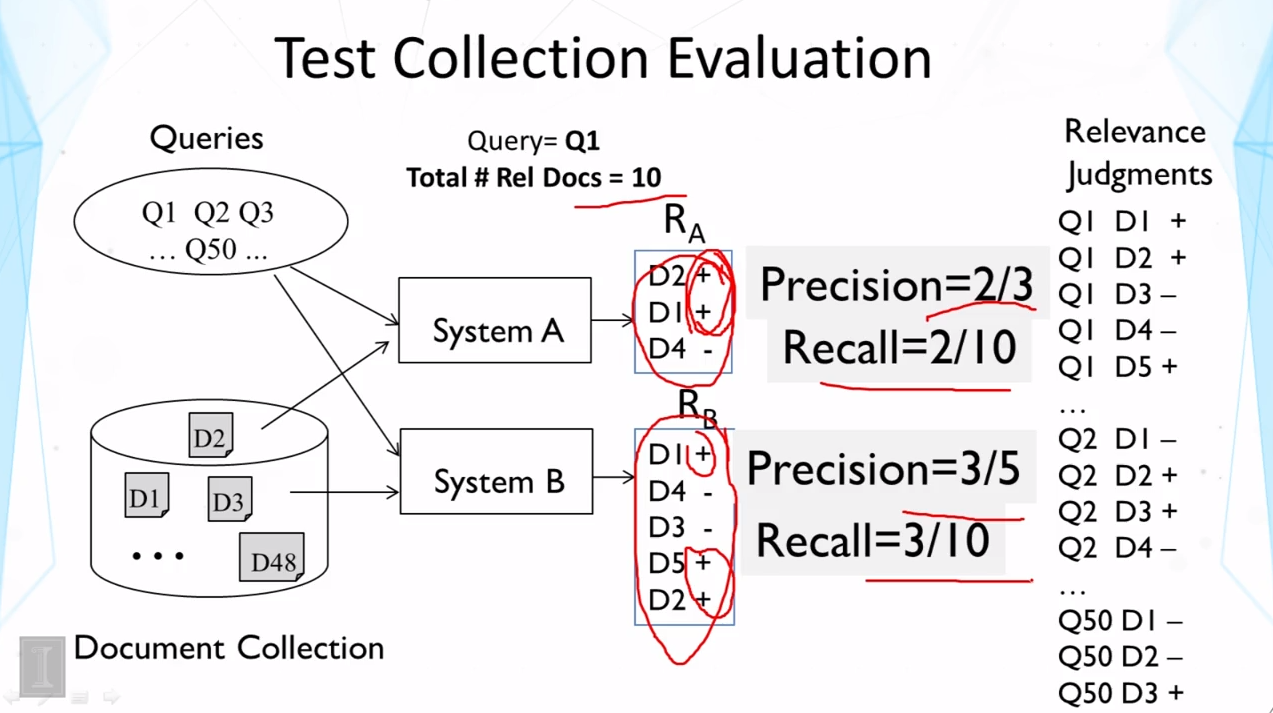$Precision=2/3 \\ Recall = 2/10$

$Precision=3/5 \\ Recall=3/10$

$Precision=\frac{结果中真正相关的文档数 }{返回的结果的文档总数}\space\space\space\space\space\space(2.1)\\Recall=\frac{结果中真正相关的文档数}{测试集中所有的相关文档数}\space\space\space\space\space\space(2.2)$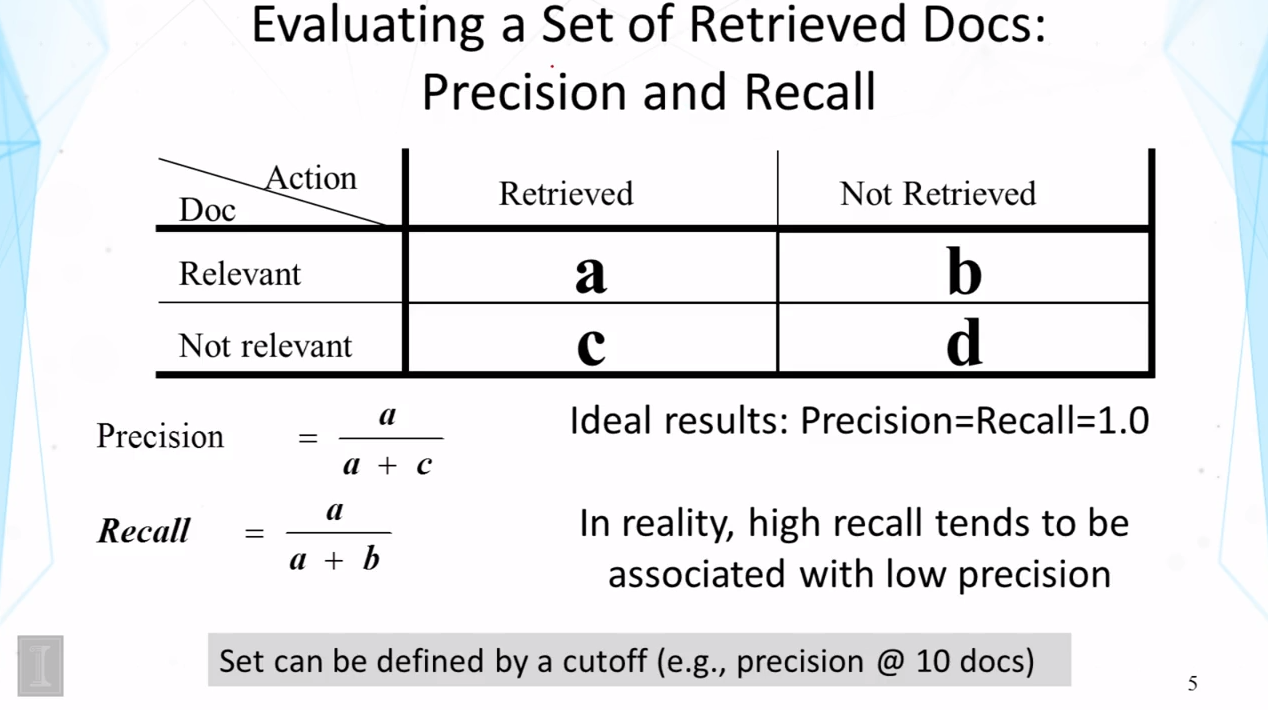### 2.连接准确度与召回率：F-Measure（Combine Precision and Recall : F-Measure）

$F_\beta=\frac{1}{\frac{\beta^2}{\beta^2+1}\frac{1}{R}+\frac{1}{\beta^2+1}\frac{1}{P}}=\frac{(\beta^2+1)P*R}{\beta^2P+R}\space\space\space\space\space\space(2.3)$

P=准确率

R=召回率

β=参数（经常被设为1）

$F_1=\frac{2PR}{P+R}$

• 当β趋近于无穷大的时候，

$\lim_{\beta\rightarrow\infty}F_\beta=\frac{\beta^2P*R}{\beta^2P}=R$

怎么理解呢？这个情况是说，β趋近于无穷大的时候，F-Measure退化成召回率R。

• 当β趋近于0的时候：

$\lim_{\beta\rightarrow0}F_\beta=\frac{P*R}{R}=P,when \space R \ne0$

当β趋近于零的时候，F-Measure退化成准确度P。

### 3.总结

• 准确率（Precision）：所有的搜索结果都是相关的吗？
• 召回率（Recall）：所有的相关文档都被搜索到了吗？
• F-Measure关联了精确率和选择率
• 如何权衡精确率和召回率的关系，取决于用户的需求

## 三、评估排名名单（Evaluating Ranked Lists）

### 1.精确率-召回率曲线（Precision-Recall Curve）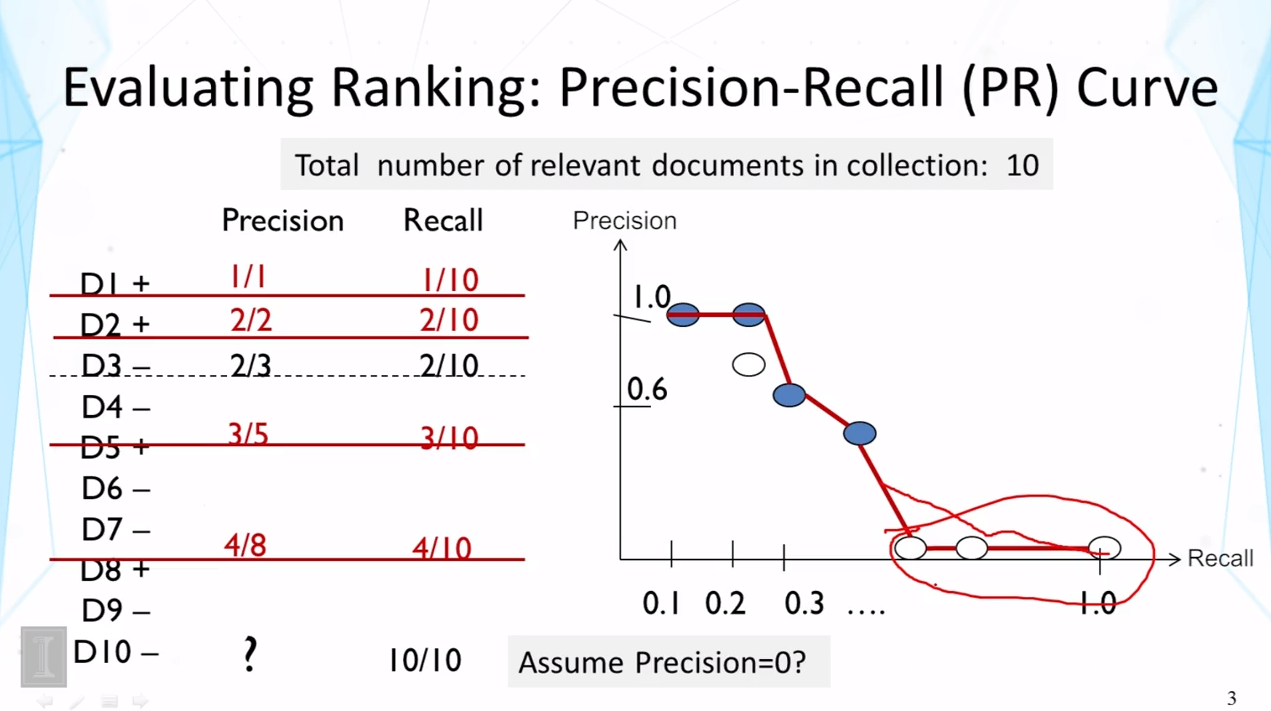### 2.如何总结一个排名名单（How to summarize a ranking）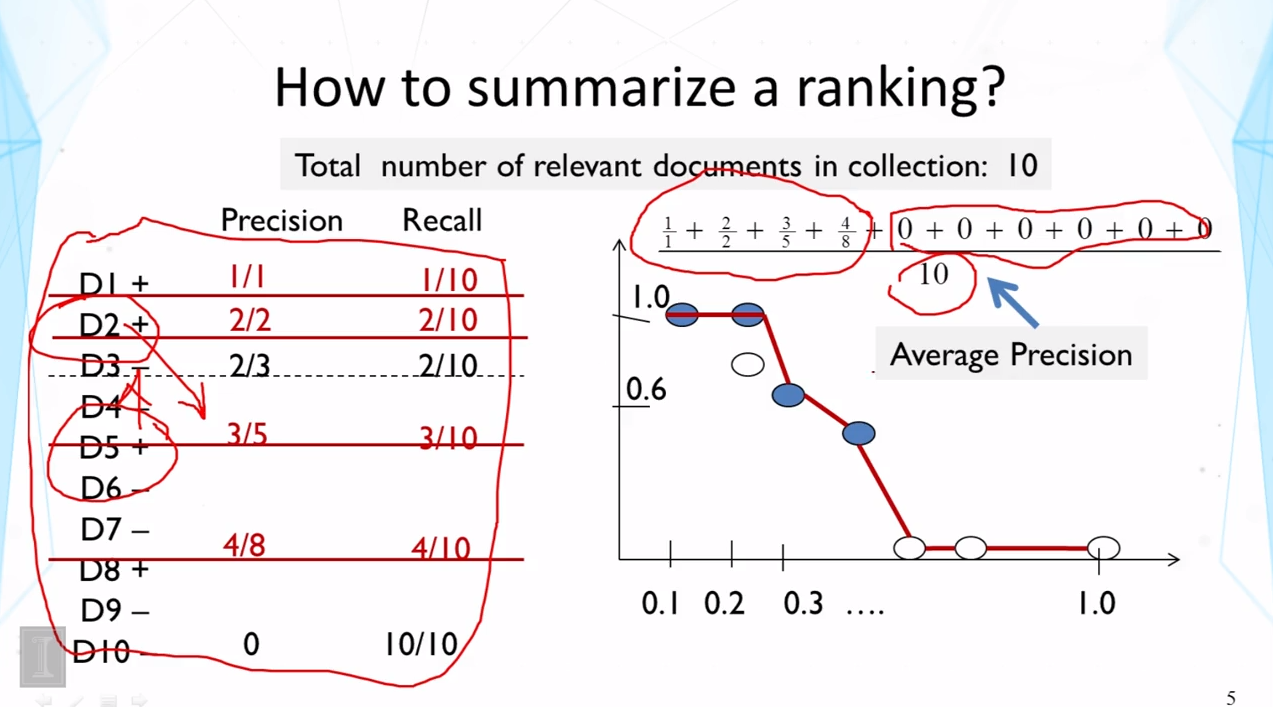$\frac{\sum_{N}{该top-N中实际相关文档数}}{测试集中相关文档的总数} \space\space\space\space\space\space ,while\space(top-N:后续还有相关文档)(3.1)$

### 3. 平均值的选取

• 平均准确率（Average Precision）
– 在正确命中相关文档的截断处进行平均值计算
– 标准化者（Normalizer）= 集合中相关文档的总号数
– 对于排名名单中每一个相关文档都很敏感
• Mean Average Precision
– MAP = 一系列Query的平均准确率的算术平均值
– gMap= 一些列Query的平均准确率的几何平均值
– 哪一个更好？

### 4.特殊情况：平均排名倒数（Special Case：Mean Reciprocal Rank）

• 平均准确率=平均排名倒数=1/r，其中r为该唯一相关文档的排名位置
• Mean Average Precision -> Mean Reciprocal Rank
• 为什么不简单地使用r？

### 5.总结

• 准确率-召回率曲线抽取出了一个排名名单的总体特征
• 一个排名名单的实际用途取决于一个用户到底会检查名单的前几位
• 平均准确率是比较两种排名方法的常规方法
– 联系了准确率和召回率
– 对于排名名单中每一个实际相关文档都敏感

## 四、多级评估（Multi-Level Judgements）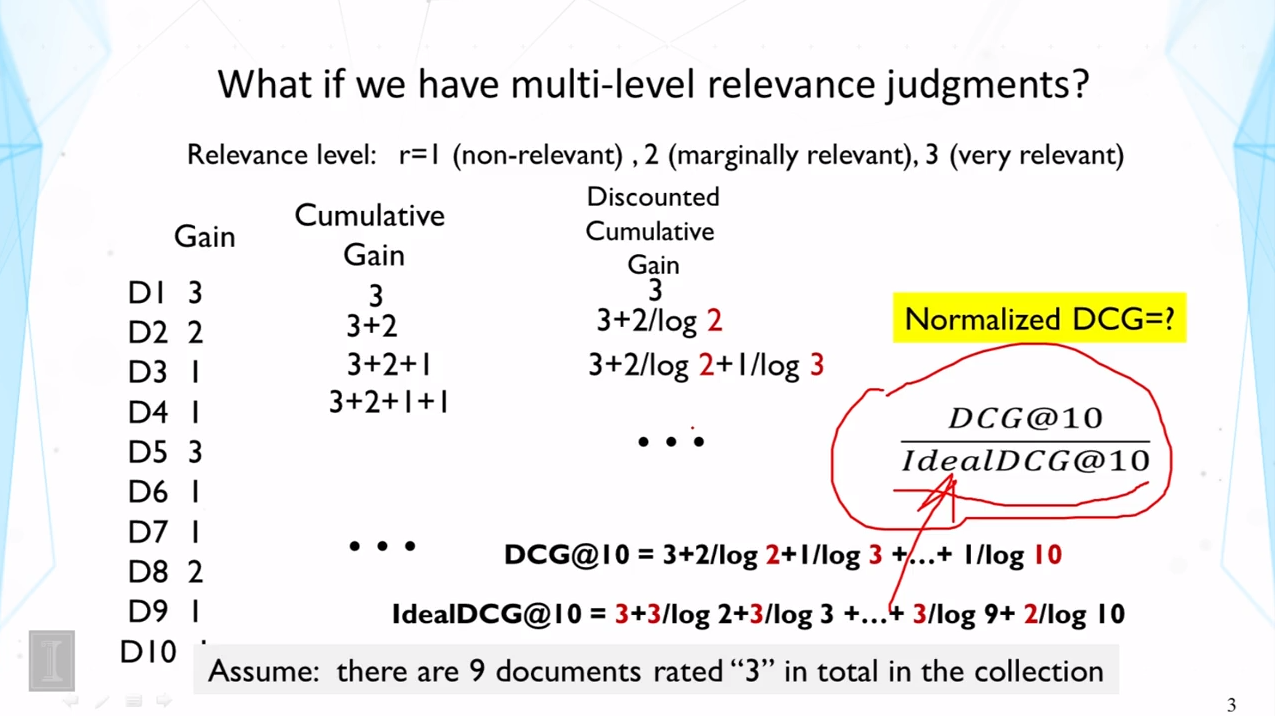1. 累积增益（Cumulative Gain） 这种方法下，直接将子集合中的预测等级相加。通过之前的讨论我们知道，这种算法并没有考虑到用户浏览不同TOP-N的可能性存在的不同。
2. 折扣累积增益（Discounted Cumulative Gain） 这种方法相对于第一种方法，考虑到了用户浏览不同TOP-N的可能性，引入了权重项logN。
Tags:

Insert math as
$${}$$